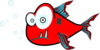Click here for the Problem Extension Worksheet version of the Problems of the Week.
Click here for an MS Word version of the Problems of the Week.
Click here for the Canadian Problem Extension Worksheet version of the Problems of the Week.
Click here for a Canadian MS Word version of the Problems of the Week.Lower Elementary:
Question: A tree frog has 4 toes on each front foot and 5 toes on each back foot. How many toes does a tree frog have in total?
Answer: 18 toes
Solution: The frog has 4 + 4 = 8 front toes and 5 + 5 = 10 back toes. That makes a total of 8 + 10 = 18 toes.Upper Elementary:
Question: Luke says he saw a piranha that was 4¾ inches long. Howard says he saw a piranha that was 4.7 inches long. Who saw the bigger piranha?
Answer: Luke
Solution: One way to solve this problem is to write both measurements as decimals. Since ¼ is equal to 0.25, ¾ must be equal to 0.25 + 0.25 + 0.25 = 0.75. That means that a piranha that is 4¾ inches long is 4.75 inches long. Since 4.75 is greater than 4.7, Luke saw the bigger piranha.Middle School:
Question: A tree is 200 feet tall. A vine growing up the trunk of the tree overtakes 10% of the tree’s remaining height each week. How high does the vine reach after 3 weeks?
Answer: 54.2 feet
Solution: On the first week, the vine grows 10% of 200 = 20 feet, leaving 200 – 20 = 180 feet untouched. On the second week, it grows 10% of 180 = 18 feet, leaving 162 feet untouched. On the third week, it grows 10% of 162 = 16.2 feet. So, in total, the vine has grown 20 + 18 + 16.2 = 54.2 feet up the tree.Algebra and Up:
Question: A biologist is monitoring a flock of toucans. The biologist determines that the number of toucans in the flock can be estimated using the function f(n) = 22 + 3n, wherein n is the number of toucan nests that the biologist counts in the flock’s territory. If the biologist estimates that there are 37 toucans in the territory, then how many nests did they count?
Answer: 5 nests
Solution: If the biologist estimates that there are 37 toucans, then f(n) = 37. Since we know f(n) = 37 and f(n) = 22 + 3n, we can reason that 37 = 22 + 3n. We can solve for n by first subtracting to get 15 = 3n and then dividing to get 5 = n. If n = 5, then the biologist counted 5 nests.